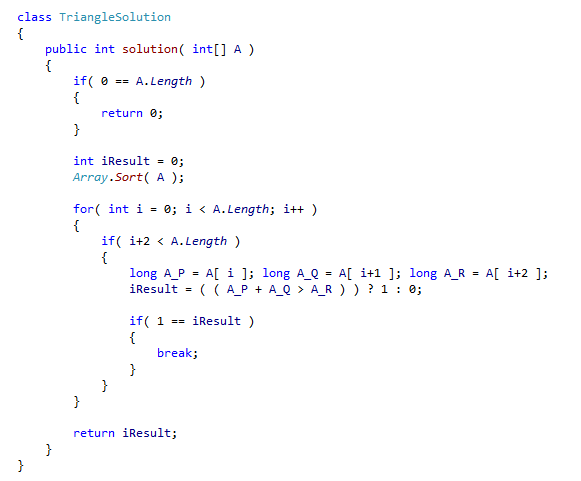### Triangle

A zero-indexed array A consisting of N integers is given. A triplet (P, Q, R) is triangular if 0 ≤ P < Q < R < N and:

• A[P] + A[Q] > A[R],
• A[Q] + A[R] > A[P],
• A[R] + A[P] > A[Q].

For example, consider array A such that:

A = 10; A = 2; A = 5; A = 1; A = 8; A = 20;

Triplet (0, 2, 4) is triangular.

Assumptions

• N is an integer within the range [0..100,000] (So guard for empty arrays)
• each element of array A is an integer within the range [−2,147,483,648..2,147,483,647] (So guard for overflow)

Language used: C#

Solution

You will be required to add numbers together here, and as they can be up to +/- 2,147,483,647 you run the risk of an overflow if you use a standard int. I used a long in my solution.

Also take in mind that although 0 ≤ P < Q < R < N, these are indices; do not confuse them for the array elements at these indices. The array may contain duplicates. After sorting the array scan for the three elements that satisfy the triangular condition. As the array is sorted you will not miss any of these elements.